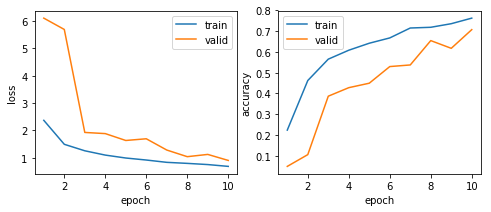### 浮世絵作者予測

Open Review Competitiontakedarts

# はじめに

TPUを使いたい場合はTensorflowでモデルを作るというのが常套手段ですが、torch_xlaを使うことでPyTorchのモデルをTPUで実行できます。

torch_xlaのgithubリポジトリ
https://github.com/pytorch/xla

このモデルのLBスコア： 0.728

# 事前準備

• 学習用ラベルデータ : ProbSpace/ukiyoe/ukiyoe-train-labels.npz
• 学習用画像データ: ProbSpace/ukiyoe/ukiyoe-train-imgs.npz

# セットアップ

OpenMPのライブラリも必要になるので、これもインストールします。

# google driveをマウント
drive.mount('/content/drive')

# torch_xla をインストール
DIST_BUCKET="gs://tpu-pytorch/wheels"
TORCH_WHEEL="torch-1.15-cp36-cp36m-linux_x86_64.whl"
TORCH_XLA_WHEEL="torch_xla-1.15-cp36-cp36m-linux_x86_64.whl"
TORCHVISION_WHEEL="torchvision-0.3.0-cp36-cp36m-linux_x86_64.whl"

!pip uninstall -y torch torchvision
!gsutil cp "$DIST_BUCKET/$TORCH_WHEEL" .
!gsutil cp "$DIST_BUCKET/$TORCH_XLA_WHEEL" .
!gsutil cp "$DIST_BUCKET/$TORCHVISION_WHEEL" .
!pip install "$TORCH_WHEEL" "$TORCH_XLA_WHEEL" "\$TORCHVISION_WHEEL"
!sudo apt-get install libomp5

# ライブラリの読み込みと乱数設定

import torch
import torch.nn as nn
import torch.optim as optim
import torchvision.models as models
import torch_xla.distributed.data_parallel as dp
import torch_xla.core.xla_model as xm
from sklearn.model_selection import train_test_split
import numpy as np
import matplotlib.pyplot as plt
import albumentations
import random
import copy
import os
import csv

#  乱数設定
RANDOM_SEED = 2020
random.seed(RANDOM_SEED)
os.environ['PYTHONHASHSEED'] = str(RANDOM_SEED)
np.random.seed(RANDOM_SEED)
torch.manual_seed(RANDOM_SEED)

print(f'random seed={RANDOM_SEED}')

# データの読み込み

データ拡張は正規化と横方向の反転のみとしました。

class UkiyoeDataset(Dataset):
def __init__(self, images, labels, train=False):
super().__init__()
self.images = images
self.labels = labels

transforms = [albumentations.Normalize(
mean=[0.721, 0.659, 0.560], std=[0.224, 0.207, 0.198], p=1)]

if train:
transforms.append(albumentations.HorizontalFlip(p=0.5))

self.transform = albumentations.Compose(transforms)

def __len__(self):
return len(self.images)

def __getitem__(self, idx):
image = np.rollaxis(self.transform(image=self.images[idx])['image'], 2, 0)
label = self.labels[idx]

return image, label

# データローダの作成
'drive/My Drive/ProbSpace/ukiyoe/ukiyoe-train-labels.npz')['arr_0']
'drive/My Drive/ProbSpace/ukiyoe/ukiyoe-train-imgs.npz')['arr_0']

train_x, valid_x, train_y, valid_y = train_test_split(
train_images, train_labels, test_size=0.2, random_state=RANDOM_SEED)

UkiyoeDataset(train_x, train_y, train=True),
batch_size=64, shuffle=True)
UkiyoeDataset(valid_x, valid_y, train=False),
batch_size=64, shuffle=False)

print(f'train data size: {len(train_x)}')
print(f'valid data size: {len(valid_x)}')

# 学習

モデルはpre-definedのResNet18にしています。

pytorchのモデルをTPUで実行するためには、学習を実行する関数（train_fun）と検証を実行する関数（valid_func）を作成し、モデルを登録したDataParallelオブジェクトにこれらの関数を渡すことで学習/検証が実行されます。

class Classifier(nn.Module):
def __init__(self, model):
super().__init__()
self.model = model
self.criterion = nn.CrossEntropyLoss()

def forward(self, images, labels):
preds = self.model(images)
loss = self.criterion(preds, labels)
accuracy = preds.max(dim=1).eq(labels).float().mean()

return loss, accuracy

loss_total = 0
accuracy_total = 0
count = 0

loss, accuracy = model(images, labels)

if optimizer is not None:
loss.backward()
xm.optimizer_step(optimizer)

loss_total += float(loss) * len(images)
accuracy_total += float(accuracy) * len(images)
count += len(images)

return np.array(
(loss_total / count, accuracy_total / count), dtype=np.float64)

optimizer = context.getattr_or('optimizer',

model.train()

model.eval()

# モデル作成
model_parallel = dp.DataParallel(Classifier(
models.resnet18(pretrained=False, num_classes=10)))
print(f'tpu devices: {model_parallel.devices}')

# 学習
log = []

for epoch in range(10):
train_loss, train_accuracy = sum(results) / len(results)

valid_loss, valid_accuracy = sum(results) / len(results)

print('[{}] train(loss/accuracy)={:.2f}/{:.2f}, valid(loss/accuracy)={:.2f}/{:.2f}'.format(
epoch + 1, train_loss, train_accuracy, valid_loss, valid_accuracy))

log.append((epoch + 1, train_loss, train_accuracy, valid_loss, valid_accuracy))

# モデルを保存
model_copy = copy.deepcopy(model_parallel.models.model).to('cpu')
torch.save(model_copy.state_dict(), 'resnet18.pth')

# 結果表示
figure = plt.figure(figsize=(8, 3))

axis.plot([x for x in log], [x for x in log], label='train')
axis.plot([x for x in log], [x for x in log], label='valid')
axis.set_xlabel('epoch')
axis.set_ylabel('loss')
axis.legend()

axis.plot([x for x in log], [x for x in log], label='train')
axis.plot([x for x in log], [x for x in log], label='valid')
axis.set_xlabel('epoch')
axis.set_ylabel('accuracy')
axis.legend()

plt.show()
tpu devices: ['xla:1', 'xla:2', 'xla:3', 'xla:4', 'xla:5', 'xla:6', 'xla:7', 'xla:8']
 train(loss/accuracy)=2.37/0.22, valid(loss/accuracy)=6.11/0.05
 train(loss/accuracy)=1.49/0.46, valid(loss/accuracy)=5.69/0.11
 train(loss/accuracy)=1.25/0.57, valid(loss/accuracy)=1.92/0.39
 train(loss/accuracy)=1.10/0.61, valid(loss/accuracy)=1.88/0.43
 train(loss/accuracy)=0.99/0.64, valid(loss/accuracy)=1.63/0.45
 train(loss/accuracy)=0.91/0.67, valid(loss/accuracy)=1.69/0.53
 train(loss/accuracy)=0.83/0.71, valid(loss/accuracy)=1.28/0.54
 train(loss/accuracy)=0.79/0.72, valid(loss/accuracy)=1.04/0.65
 train(loss/accuracy)=0.75/0.74, valid(loss/accuracy)=1.12/0.62
 train(loss/accuracy)=0.68/0.76, valid(loss/accuracy)=0.90/0.71
/usr/local/lib/python3.6/dist-packages/torch_xla_py/__init__.py:2: UserWarning: torch_xla_py has been restructured to torch_xla and it will be removed soon, please call the submodules in torch_xla directly.
warnings.warn('torch_xla_py has been restructured to torch_xla and it will be removed soon, '# リーダーボードのスコアを予測

テストデータに含まれる各クラスの割合を、あらかじめ調べておきました（すべて同じクラスにしたsubmissionを提出すると割合が得られます）。 テストデータに含まえるクラス$i$の割合が$p_i$、クラス$i$の検証データの再現率が$r_i$の場合、リーダーボードの予想スコアを$\sum p_i r_i$としています。

# モデル読み込み
model = models.resnet18(pretrained=False, num_classes=10)

# 推論
results = [ * 10 for _ in range(10)]

device = xm.xla_device()
model = model.to(device)
model.eval()

preds = model(images.to(device)).max(dim=1).cpu()

for label, pred in zip(labels, preds):
results[label][pred] += 1

true_pos_total = 0
false_pos_total = 0
false_neg_total = 0
fscore_total = 0
lbscore_total = 0

# 表示
test_dists = [ # テストデータに含まれる各クラスの割合
0.181, 0.209, 0.093, 0.068, 0.113, 0.063, 0.116, 0.048, 0.058, 0.050]

print('-- predictions (L:Labels, P:Predictions)')
print('   |{}'.format('|'.join(f'P={i}' for i in range(10))))
for i in range(10):
print('L={}|{}'.format(i, '|'.join(f'{r:3d}' for r in results[i])))

print()
print('-- scores')
print('   |count|precision|recall|f-score')
for i in range(10):
true_pos = results[i][i]
false_neg = sum(results[i]) - true_pos
false_pos = sum(results[j][i] for j in range(10)) - true_pos
precision = true_pos / (true_pos + false_pos)
recall = true_pos / (true_pos + false_neg)
fscore = (2 * precision * recall) / (precision + recall)

true_pos_total += true_pos
false_pos_total += false_pos
false_neg_total += false_neg
fscore_total += fscore
lbscore_total += test_dists[i] * recall

print('L={}|  {:3d}|   {:.4f}|{:.4f}| {:.4f}'.format(
i, sum(results[i]), precision, recall, fscore))

print()
print('accuracy       = {:.4f}'.format(true_pos_total / len(valid_x)))
print('f-score(micro) = {:.4f}'.format(
(2 * true_pos_total) / (2 * true_pos_total + false_pos_total + false_neg_total)))
print('f-score(macro) = {:.4f}'.format(fscore_total / 10))
print('estimated LB   = {:.4f}'.format(lbscore_total))
-- predictions (L:Labels, P:Predictions)
|P=0|P=1|P=2|P=3|P=4|P=5|P=6|P=7|P=8|P=9
L=0|120|  4|  0|  0|  0|  4|  1|  0|  0|  0
L=1| 10| 88|  7|  3|  6|  1| 18|  0|  0|  1
L=2|  0|  0| 43|  0|  3|  2|  3|  1|  5|  0
L=3|  0|  8|  1| 18|  4|  0|  3|  5|  0|  0
L=4|  0|  4|  9|  0| 54|  0|  5|  0|  4|  0
L=5|  8|  4|  1|  0|  4| 18|  0|  0|  0|  0
L=6|  0|  0|  9|  0|  1|  0| 73|  0|  1|  0
L=7|  0|  5|  3|  3|  0|  0|  3| 16|  0|  0
L=8|  0|  4|  7|  0|  8|  1|  1|  0| 14|  0
L=9|  0|  2|  0|  0|  0|  0|  1|  0|  0| 10

-- scores
|count|precision|recall|f-score
L=0|  129|   0.8696|0.9302| 0.8989
L=1|  134|   0.7395|0.6567| 0.6957
L=2|   57|   0.5375|0.7544| 0.6277
L=3|   39|   0.7500|0.4615| 0.5714
L=4|   76|   0.6750|0.7105| 0.6923
L=5|   35|   0.6923|0.5143| 0.5902
L=6|   84|   0.6759|0.8690| 0.7604
L=7|   30|   0.7273|0.5333| 0.6154
L=8|   35|   0.5833|0.4000| 0.4746
L=9|   13|   0.9091|0.7692| 0.8333

accuracy       = 0.7184
f-score(micro) = 0.7184
f-score(macro) = 0.6760
estimated LB   = 0.7079


# 提出データの作成

テストデータの推論結果から提出ファイルを作成します。

# データ読み込み
'drive/My Drive/ProbSpace/ukiyoe/ukiyoe-test-imgs.npz')['arr_0']
test_labels = np.zeros((test_images.shape, 1), dtype=np.int64)

UkiyoeDataset(test_images, test_labels, train=False),
batch_size=64, shuffle=False)

# 推論
predictions = []
model.eval()

preds = model(images.to(device)).max(dim=1).cpu()

predictions.extend(int(x) for x in preds)

# results
classes =  * 10
dists = [int(round(d * len(predictions))) for d in test_dists]
error = 0

for p in predictions:
classes[p] += 1

print("-- number of predictions")
for i, (c, d) in enumerate(zip(classes, dists)):
error += abs(c - d)
print(f'class-{i}: {c:3d} (actual={d})')

# 提出ファイル作成
with open('submission.csv', 'w') as writer:
csv_writer = csv.writer(writer)
csv_writer.writerow(('id', 'y'))
csv_writer.writerows((i + 1, x) for i, x in enumerate(predictions))
-- number of predictions
class-0:  76 (actual=72)
class-1:  82 (actual=83)
class-2:  37 (actual=37)
class-3:  22 (actual=27)
class-4:  43 (actual=45)
class-5:  27 (actual=25)
class-6:  60 (actual=46)
class-7:  14 (actual=19)
class-8:  22 (actual=23)
class-9:  14 (actual=20)


#### 添付データ

• ukiyoe-tpu.ipynb?X-Amz-Expires=10800&X-Amz-Date=20231209T154003Z&X-Amz-Algorithm=AWS4-HMAC-SHA256&X-Amz-Credential=AKIAIP7GCBGMWPMZ42PQ
•###### camaro

また、DataParallelを使っているとエラーが出た際に毎回カーネルが落ちてしまうのですが、そういうものでしょうか？ 上手いデバグのやり方があれば教えていただけると助かりますm(__)m###### camaro###### camaro###### takedarts

> camaroさん
Classifierクラスは作らなくても問題ありません。関数perform内の処理を以下のコードに変更しても問題なく実行できました。

    #loss, accuracy = model(images, labels)
preds = model.model(images)
loss = nn.functional.cross_entropy(preds, labels)
accuracy = preds.max(dim=1).eq(labels).float().mean()